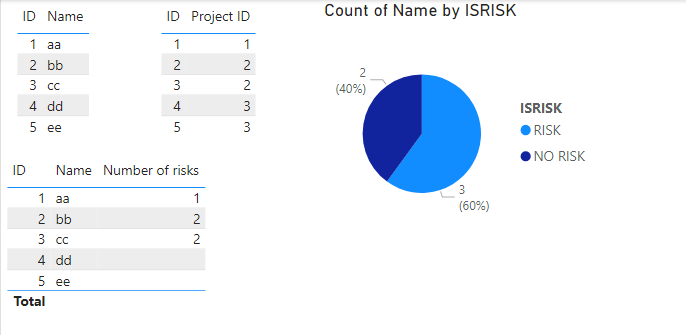cancel
Showing results for
Did you mean:Regular Visitor

## Making a risk report from MS Project Online - creating visuals

I am a new user and am trying to create a risk report on PowerBI using information about projects which has been imported from MS Project Online.

1. I want to calculate the number of risks per project and show this in a visual. I think I need to add an extra column in the underlying data tables using a fornula to calculate this. However, I'm unsure whether this should be created in the Risks table or the Projects table and what formula to use. Each Project has a unique ProjectID and this is the common key between tables. Each Risk also has a unique RiskID but multiple risks can be linked to each project and some have no risks attached at all.

2. I would then like to create a donut chart which shows the total number of projects available but split by whether or not they have any risks attached. I think I can use the information from step one and then when I know whether a project has a risk number of 0 or anything more, I should turn this in to a binary figure. However, I am unsure how to create a formula in the data tables to do so.

Any help would be much appreciated.

1 ACCEPTED SOLUTIONCommunity Support

Hi @Charday ,

(1)Create a measure to calculate the number of risks per project.

``Number of risks = CALCULATE(COUNT(Risk[ID]),FILTER(ALL(Risk),Risk[Project ID] = MAX(Project[ID])))``

(2) Create a calculated column to determine whether the project is at risk

``ISRISK = IF([Number of risks]>0,"RISK","NO RISK")``

(3) Final outputBest Regards,

Gallen Luo

If this post helps, then please consider Accept it as the solution to help the other members find it more quickly.Community Support

Hi @Charday ,

(1)Create a measure to calculate the number of risks per project.

``Number of risks = CALCULATE(COUNT(Risk[ID]),FILTER(ALL(Risk),Risk[Project ID] = MAX(Project[ID])))``

(2) Create a calculated column to determine whether the project is at risk

``ISRISK = IF([Number of risks]>0,"RISK","NO RISK")``

(3) Final outputBest Regards,

Gallen Luo

If this post helps, then please consider Accept it as the solution to help the other members find it more quickly.